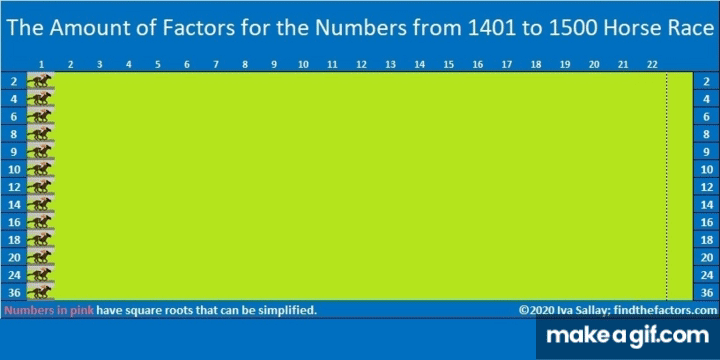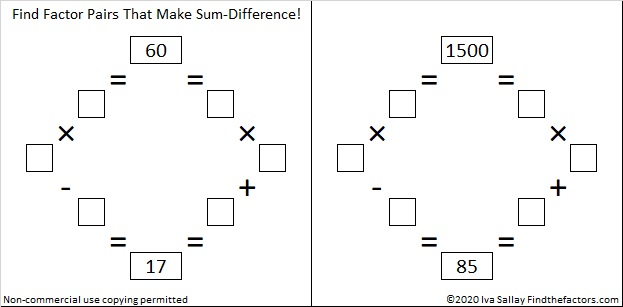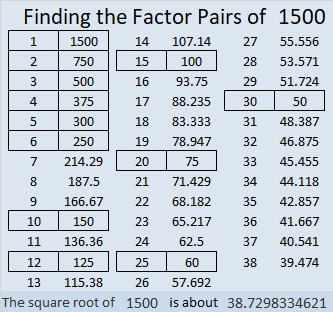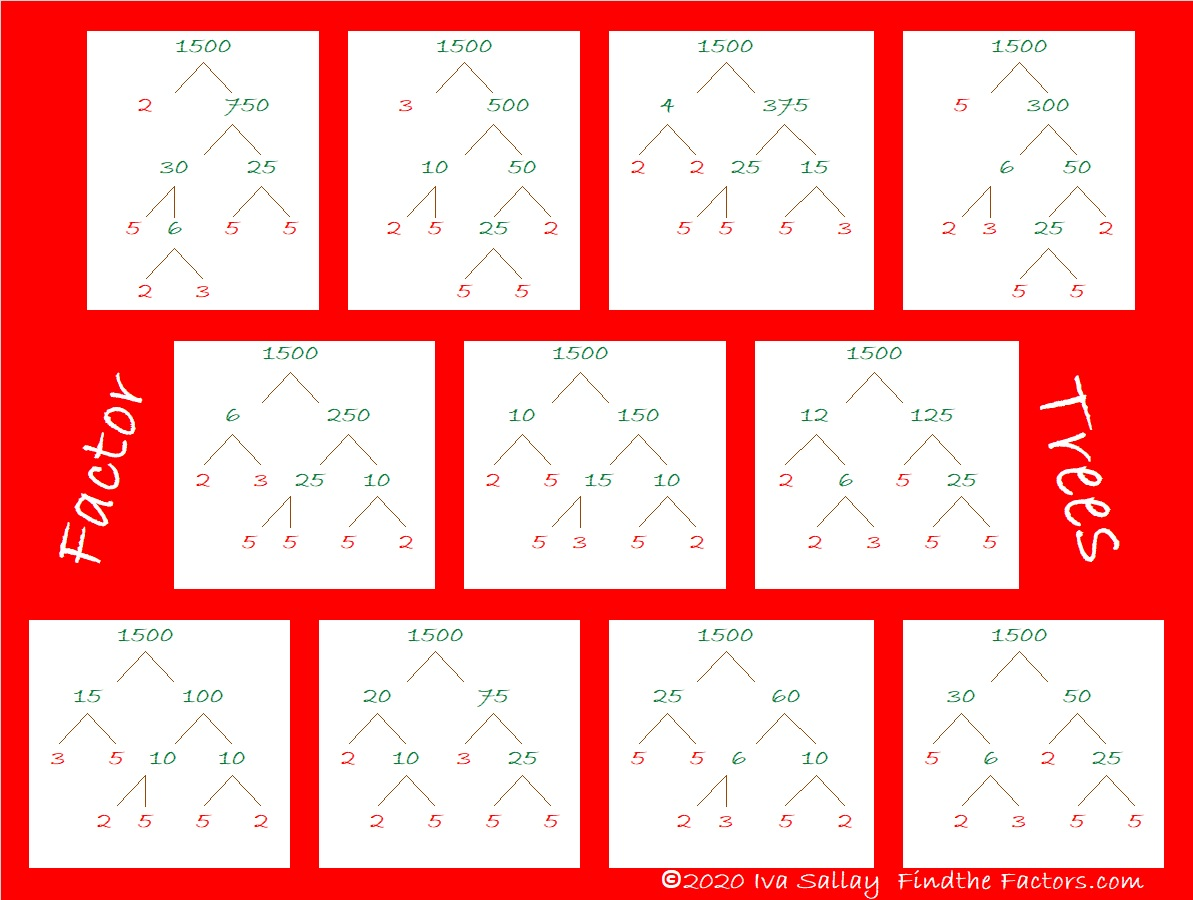# Celebrating 1500 with a Horse Race and Much More!

Writing 1500 posts is quite a milestone. I’ll begin the celebration with an exciting horse race! Let me explain:

Each prime number has exactly 2 factors. Every composite number between 1401 and 1500 has somewhere between 4 and 36 factors. Which quantity of factors do you think will appear most often for these numbers? Pick that amount as your pony and see how far it gets in this horse race!make science GIFs like this at MakeaGif

Did the race results surprise you? They surprised me!

### Prime Factorization for numbers from 1401 to 1500:

Here’s a chart showing the prime factorization of all those numbers and the amount of factors each number has. Numbers in pink have exponents in their prime factorization so their square roots can be simplified:### Today’s Puzzles:

Let’s continue the celebration with a puzzle: 1500 has 12 different factor pairs. One of those pairs adds up to 85 and one of them subtracts to give 85. Can you find those factor pairs that make sum-difference and write them in the puzzle? You can look at all of the factor pairs of 1500 in the graphic after the puzzle, but the second puzzle is really just the first puzzle in disguise. So try solving that easier puzzle first.### Factors of 1500:

• 1500 is a composite number.
• Prime factorization: 1500 = 2 × 2 × 3 × 5 × 5 × 5, which can be written 1500 = 2² × 3 × 5³
• 1500 has at least one exponent greater than 1 in its prime factorization so √1500 can be simplified. Taking the factor pair from the factor pair table below with the largest square number factor, we get √1500 = (√100)(√15) = 10√15
• The exponents in the prime factorization are 2, 1, and 3. Adding one to each exponent and multiplying we get (2 + 1)(1 + 1)(3 + 1) = 3 × 2 × 4 = 24. Therefore 1500 has exactly 24 factors.
• The factors of 1500 are outlined with their factor pair partners in the graphic below.### A Forest of Factor Trees:

Take a few minutes to hike in this forest featuring a few of the MANY possible factor trees for 1500. Celebrate each tree’s uniqueness!### Other Facts to Celebrate about 1500:

Oeis.org tells us that  (5+1) × (5+5) × (5+0) × (5+0) = 1500.

1500 is the hypotenuse of THREE Pythagorean triples:
420-1440-1500, which is (7-24-25) times 60,
528-1404-1500, which is 12 times (44-117-225),
900-1200-1500, which is (3-4-5) times 300.